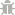Light Mode
Rate us!# pressure conversion

For pressure conversion, enter the value that requires transformation to the other unit in the “From” field, after that click or tap on the unit in which you want to turn that value in the “To” field. The conversion will be made and results will be shown immediately.

From: Newton/sq.meter
To: Newton/sq.meter

At Small SEO Tools, we are very much able to do your conversions with ease. Just like using a pressure calculator, you need not to sweat it to change one unit of pressure to another. Our website has an area for pressure unit conversion without any difficulty.

Furthermore, it equally performs the function of a water pressure calculator. Without asking you for the physics formula used for such calculations, conversions get done within a second. Knowing the pascal conversion at your fingertips might be arduous at the time of need.

Note however that the Pascal unit simply refers to the SI Unit of Pressure and stress. Just as well, atmospheric pressure, often regarded as air pressure is the force which is exerted on a surface by the air above it when gravity pulls it towards the earth. Air pressure is basically the sea level air pressure of 101.325kPa.

All necessary details are easily calculated with our efficient pressure conversion calculator made available for your use online.

## Take Note Of Units Of Pressure For Your Conversions

It is a fact that it might get really confusing when you’re required to alternate between pressure units; from bars (b) to kilopascals (kPa) to Pascal to atm to torr (Torr) and the measurement of each unit. Note that the common units used to quantify pressure include atmosphere (atm), Torr, Pascal (Pa), bar and millibar (mbar), pounds per square inch (psi)’

Well, of course, you might be very familiar already with the standard atmospheric pressure. Also, the fact that pressure is merely the amount of force that is applied to a surface where force is being distributed should not be too strange to you.

In addition, the standard atmospheric pressure which is a unit of pressure measured at 1013.25 mbar(101.325kPa) can be calculated on this Pressure Calculator.

However, when it comes to Pressure Conversion to various units, you might require some quick help. This is why we have made available with our free and easy-to-use pressure calculator.

### How to Use Pressure Conversion Calculator

The process of our Pressure Conversion calculator is very simple to use, as well as free and basic for every user. For instance, a conversion from atm to Pascal which can well be done manually need not take much effort anymore. By following the steps given below, you instantly see that a conversion from 1atm to Pa is 101325.

So, you might now ask, how do I use the online Pressure Conversion correctly? Well, you simply have to follow the following steps:

1. Step 1: Visit: https://smallseotools.com/unit-converter/
2. Step 2: Select “Pressure & Stress Converter” from the list of tools for pressure conversion.
3. Step 3: Select the unit you want to convert from the options which indicate “From”
4. Step 4: Write the number or amount of pressure you want to convert.
5. Step 5: Make a selection of the unit for the “To” field, from the list of units. This will be the unit in which you want to convert the value.
6. The correct figure automatically appears in the box